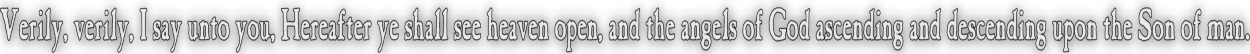None:
Polyps:
Strongs:CreationismLike Unto The Most High? Now in outr associations of bows we could state that with the four earthy elements {d,e,f,g} we have the associations with static triples to unity of; g = {d,e,f} f = {d,e,g} e = {d,f,g} d = {e,f,g} And g is associated with T(d) ∩ T(e) ∩ T(f) And T(g) is associated with (d ∪ e ∪ f) We may now replace our map T from {d,e,f,g} to {{e,f,g},{d,f,g},{d,e,g},{e,f,g}} with a function that sends a set to its complement. Then we may recover demorgans laws on triples! For we now have; g = (dc ∩ ec ∩ fc) = {d,e,f}c Where g is associated with the complement of the triple {d,e,f} This may not seem like much, for the operation is not binary - however the dialectic balances of the black horse are such that the unity element is "absorbed" on either scale of the balances: So we may synthesise from {d,f,g} => {d,e,g} <= {e,f,g} and absorb (lighten the scales) of the unity element ('f' here) to synthesise {d,g} => {d,e,g} <= {e,g} Now, again this seems somewhat unimpressive, but in absorbing unity we have efectively sent the unity element to the empty set {} as if it were "killed" or "hurt". Now, what do we obtain from our demorgans laws? If we set say, 'd' as unity and map it onto {} then, dc = {}c = {d,e,f,g} = {e,f,g} g = (dc ∩ ec ∩ fc) = {d,e,f,g} ∩{d,f,g} ∩{d,e,g} = {e,f,g} ∩{f,g} ∩{e,g} = g Or, (d ∪ e ∪ f) = (e ∪ f) and therefore g = (e ∪ f)c Which is remarkably similar to the K4 addition operation of (A v B )c However, if you missed it the choice of "g" was arbitrary as "wine" and we now see that on sending unity to the empty set we have a substitute operation for the bow in the form of an similarity to K4. So with d => {} e = (f ∪g)c f = (e ∪g)c g = (e ∪ f)c However g*g = gc = {d,e,f} = {e,f} and not the empty set 'd' or {}. Oooh, so close. Now the induced multiplication of C3 on the triple from frobenius may very well still be in place, but we should require a sufficent condition to assure that g*g = 0 or {} in some way before we muddy the waters with a multiplication before any addition is defined. If we actually have T(g) = gc = {d,e,f} we may substitute for T(g) = g and obtain g*g = g*{d,e,f} = (g ∪{d,e,f})c = {d,e,f,g}c = {} as desired. But then {e,f} = {}. unless g*T(g) is never equal to g*g. Note that T(g)*T(g) = g. and g*g = T(g) If we replace our wine g with T(g) in e = (f ∪g)c We would have e = fc ∩ g = g which is not adequate. If we replace one side as say, with T(e) then T({f,g}) = (f ∪g)c = {d,e} => {e} Which is much more useful. Then we would expect T(g) = g*g = {d,e,f} And then in order for associativity to hold, we would not require g*(g*g) but g*T(g) = {}. But note T(x) = (x ∪ {})c So equating x to T(x) would assert that {} is similar to an identity, But we do not have g*g = {} but g*g = T(g). There is no simple answer! Of course we should not limit ourselves to merely these earthy elements; forming triples in the left handed octal; we should also use the earthy elements of the left hand that correspond to right handed subgroups. Continue To Next Page Return To Section Start Return To Previous Page'